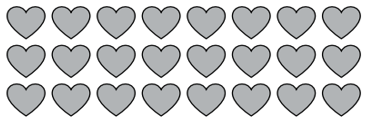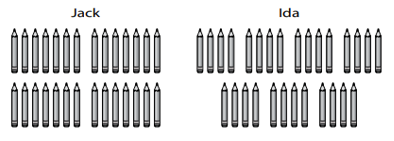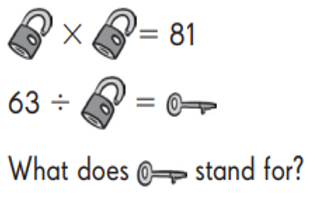RB 3rd Meaning of Division
starstarstarstarstarstarstarstarstarstar
by SAMANTHA AYODELE
| 12 Questions
1
1
What is the missing number?
? / 7 = 40 / 8
2
1
Ben has 20 balls and 4 bags. He puts the same number of balls into each bag. How many balls does Ben put into each bag? How many balls does Ben put into each bag? Write a division equation and draw and array.3
1
Which 2 division equations are shown by the array? Select all the correct answers.
A. 24/8 = 3
B. 24/6 = 4
C. 24/3 = 8
D. 24/4 = 6
E. 24/8 = 16
4
1
Amelia makes 48 paper cranes for her teachers. She gives each teacher a string of 8 paper cranes. How many teachers does she give paper cranes to?
5
1
Steve gives away 72 cards. He gives an equal number of cards to each of his 8 friends. How many cards does Stevie give to each friend? Show your work.
6
1
There are 80 students going on a class trip. Each van seats 10 students. How many vans are needed for the class trip? Explain your thinking.7
1
Jack and Ida have the same number of pencils. They each divide their pencils into equal groups as shown above. Write the equations that matches Jack and Ida's statements.
Jack's picture shows ______ divided by 4 groups of pencils = _______ pencils in each group.
Ida's picture shows ______ divided by _______ groups of pencils = _______ pencils in each group.
8
1
Which problem can be solved using the division equation 12/4=___?
Jun puts 12 stickers on each page. How many stickers does Jun put on 4 pages?
Kate has 12 stickers. She gives 4 stickers to a friend. How many stickers does Kate have now?
Max puts 12 stickers on one page. He puts 4 stickers on anoter page. How many stickers does Max put on the pages in all?
Ana has 12 stickers. She puts an equal number of stickers on each of 4 pages. How many stickers does Ana put on each page?

9
1
There are 16 flowers to be planted in Emily's garden. There are 2 flower pots. If there are an equal number of flowers for each pot, how many flowers will be planted in each pot?
10
1
Divide 25 tens by 5. What is the answer?
a. 5 ones
b. 50 ones
c. 15 tens
d. 50 tens11
1
What does the key stand for?
12
1
What do you get when you divide 23 by 3?
A. 2 remainder 7
B. 7 remainder 2
C. 7 remainder 3
D. 2 remainder 3